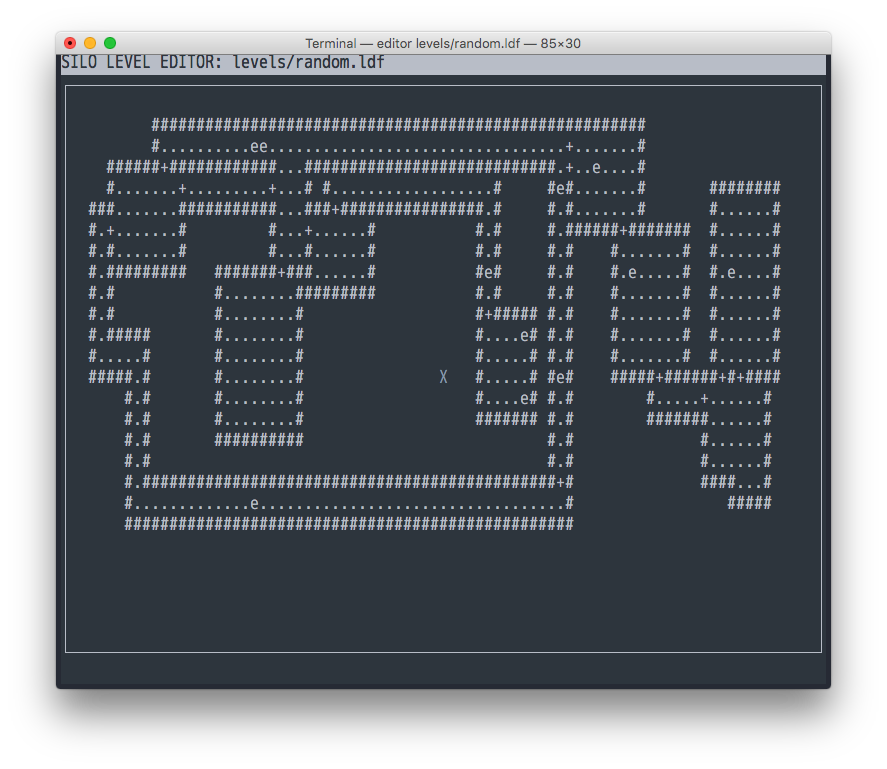After generating a few good looking random dungeons, I was puzzled with randomly placing mobs on resulting maps. To make it fair (and fun) for the player mobs should be evenly distributed.The obvious idea was to pick coordinates randomly within the rectangular map boundaries, and then place mobs if they have floor underneath them. But this way I lose control of the number of mobs and risk having a chance of not placing any mobs at all. Plus, dungeons with bigger surface area would get more mobs - which sounds somewhat realistic, but not entirely what I was aiming for.

I could improve on the above by rerunning enemy placement multiple times and select the most favorable outcome - but the solution would be rather naive.

To have control over the number of mobs I decided to place them as I generate the rooms of the dungeon. There’s a trick one can use to get a random element with equal probability distribution from a sequence of an unknown size:

``````import random

def get_random_element(sequence):
"""Select a random element from a sequence of an unknown size."""
selected = None
for k, element in enumerate(sequence):
if random.randint(0, k) == 0:
selected = element
return selected
``````

With each iteration the chance of the current element to become a selected item is 1 divided by number of elements seen so far. Indeed, a probability of an element being selected out of a 4-item sequence:

``````1 * (1 - 1/2) * (1 - 1/3) * (1 - 1/4) = 1/2 * 2/3 * 3/4 = 6/30 = 1/4
``````

Now all I had to do is to modify this to account for multiple mob placement. Here’s a generalized function above which accounts for selecting `n` elements from the sequence with even distribution.

``````import random

def get_random_element(sequence, n):
"""Select n random elements from a sequence of an unknown size."""
selected = [None for _ in range(n)]
for k, element in enumerate(sequence):
for i in range(n):
if random.randint(0, k) == 0:
selected[i] = element
return selected
``````

I incorporated logic above into the room generation code, accounted for duplicates, and ended up with decent distribution results.